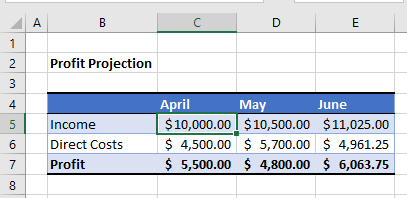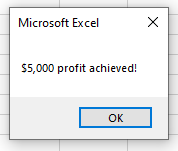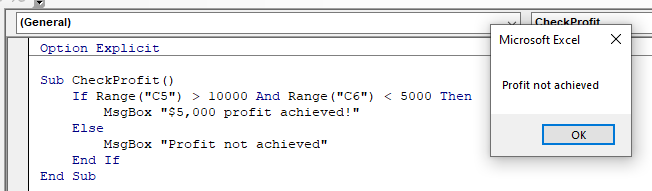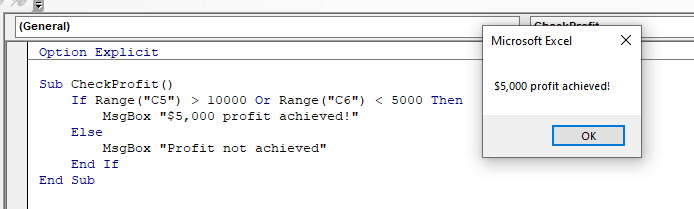# VBA If – And, Or, Not

This article will demonstrate how to use the VBA If statement with And, Or and  Not.

When we us an IF statement in Excel VBA, the statement will execute a line of code if the condition you are testing is true.

• We can use AND statement and OR statements in conjunction with IF statements to test for more than one condition and direct the code accordingly.
• We can also use a NOT statement with an IF statement to check if the condition is NOT true – it basically is the inverse of the IF statement when used alone.

## IF…AND

We can use the IF…AND combination of logical operators when we wish to test for more than one condition where all the conditions need to be true for the next line of code to execute.

For example, consider the following sheet:To check if the Profit is over \$5,000, we can run the following macro:

``````Sub CheckProfit()
If Range("C5") >= 10000 And Range("C6") < 5000 Then
MsgBox "\$5,000 profit achieved!"
Else
Msgbox "Profit not achieved!"
End If
End Sub``````

This macro will check that the cell C5 is greater or equal to \$10,000 AND check that the cell B6 is less than \$5,000. If these conditions are BOTH true, it will show the message box.If we amend the macro to check if C5 is just greater than \$10,000, then the profit would not be achieved!## IF…OR

We can use the IF…OR combination of logical operators when we wish to test for more than one condition where only one of the conditions needs to be true for the next line of code to execute.

The format for this is almost identical to the IF…AND example above.

``````Sub CheckProfit()
If Range("C5") > 10000 Or Range("C6") < 5000 Then
MsgBox "\$5,000 profit achieved!"
Else
Msgbox "Profit not achieved!"
End If
End Sub``````

However, with this macro, because we are using an IF …OR statement, only one of the conditions needs to be true.## IF NOT…

IF..NOT  changes the IF statement around – it will check to see if the condition is NOT true rather than checking to see if the condition is true.

``````Sub CheckProfit()
If NOT Range("C5")< 10000 Or Range("C6") < 5000 Then
MsgBox "\$5,000 profit achieved!"
Else
Msgbox "Profit not achieved!"
End If
End Sub``````

In this example above, the IF statement is checking to see if the value in C5 is NOT smaller than 10000.

Therefore this line of code:

``IF Range("C5") > 10000``

and this this line of code:

``IF NOT Range("C5") < 10000``

are testing for the same thing!